# Deep SORT论文阅读笔记¶

## 1. 简介¶

Simple Online and Realtime Tracking(SORT)是一个非常简单、有效、实用的多目标跟踪算法。在SORT中，仅仅通过IOU来进行匹配虽然速度非常快，但是ID switch依然非常大。

## 2. 方法¶

### 2.1 状态估计¶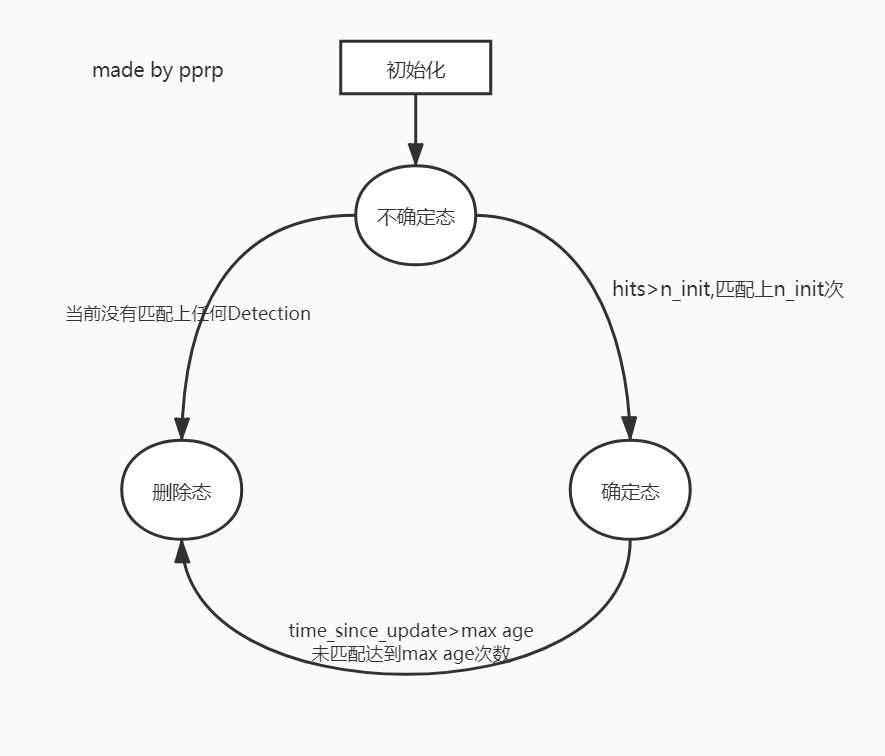### 2.2 匹配问题¶

Assignment Problem指派或者匹配问题，在这里主要是匹配轨迹Track和观测结果Detection。这种匹配问题经常是使用匈牙利算法(或者KM算法)来解决，该算法求解对象是一个代价矩阵，所以首先讨论一下如何求代价矩阵：

• 使用平方马氏距离来度量Track和Detection之间的距离，由于两者使用的是高斯分布来进行表示的，很适合使用马氏距离来度量两个分布之间的距离。马氏距离又称为协方差距离，是一种有效计算两个未知样本集相似度的方法，所以在这里度量Track和Detection的匹配程度。
d^{(1)}(i,j)=(d_j-y_i)^TS_i^{-1}(d_j-y_i)
b_{i,j}^{(1)}=1[d^{(1)}(i,j)\le t^{(1)}]

$d_j$代表第j个detection，$y_i$代表第i个track，$S_i^{-1}$代表d和y的协方差。

• 使用cosine距离来度量表观特征之间的距离，reid模型抽出得到一个128维的向量，使用余弦距离来进行比对：
d^{(2)}(i,j)=min\{1-r_j^Tr_k^{(i)}|r_{k}^{(i)}\in R_i\}

$r_j^Tr_k^{(i)}$计算的是余弦相似度，而余弦距离=1-余弦相似度，通过cosine距离来度量track的表观特征和detection对应的表观特征，来更加准确地预测ID。SORT中仅仅用运动信息进行匹配会导致ID Switch比较严重，引入外观模型+级联匹配可以缓解这个问题。

b_{i,j}^{(2)}=1[d^{(2)}(i,j)\le t^{(2)}]

• 综合匹配度是通过运动模型和外观模型的加权得到的
c_{i,j}=\lambda d^{(1)}(i,j)+(1-\lambda)d^{(2)}(i,j)

b_{i,j}=\prod_{m=1}^2b_{i,j}^{(m)}

$b_{i,j}$也是指示器，只有$b_{i,j}=1$的时候才会被人为初步匹配上。

### 2.3 级联匹配¶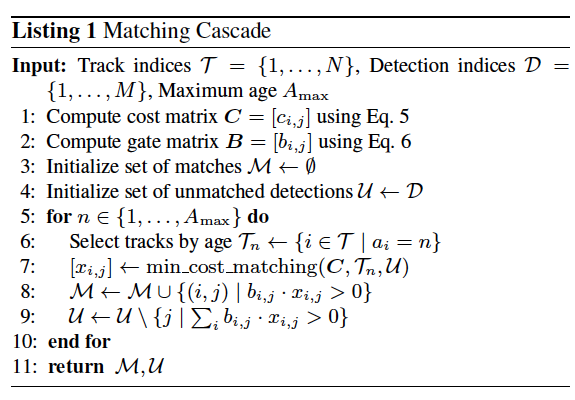# 1. 分配track_indices和detection_indices
if track_indices is None:
track_indices = list(range(len(tracks)))

if detection_indices is None:
detection_indices = list(range(len(detections)))

unmatched_detections = detection_indices

matches = []
# cascade depth = max age 默认为70
if len(unmatched_detections) == 0:  # No detections left
break

track_indices_l = [
k for k in track_indices
if tracks[k].time_since_update == 1 + level
]
if len(track_indices_l) == 0:  # Nothing to match at this level
continue

# 2. 级联匹配核心内容就是这个函数
matches_l, _, unmatched_detections = \
min_cost_matching(  # max_distance=0.2
distance_metric, max_distance, tracks, detections,
track_indices_l, unmatched_detections)
matches += matches_l
unmatched_tracks = list(set(track_indices) - set(k for k, _ in matches))
return matches, unmatched_tracks, unmatched_detections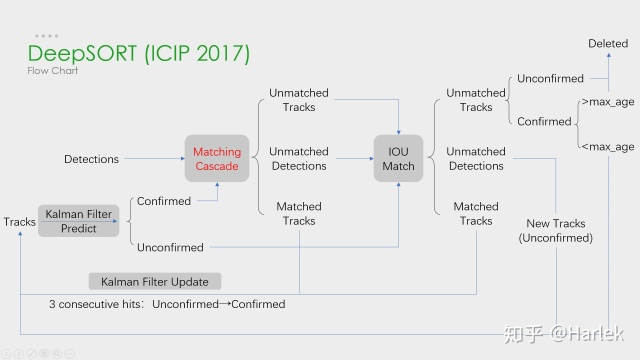### 2.4 表观特征¶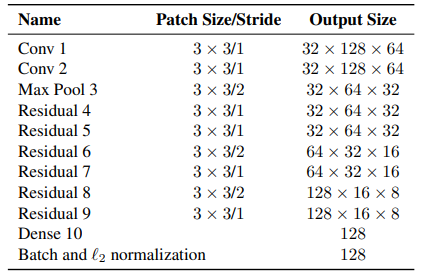## 3. 实验¶

FP很多，主要是由于Detection和Max age过大导致的。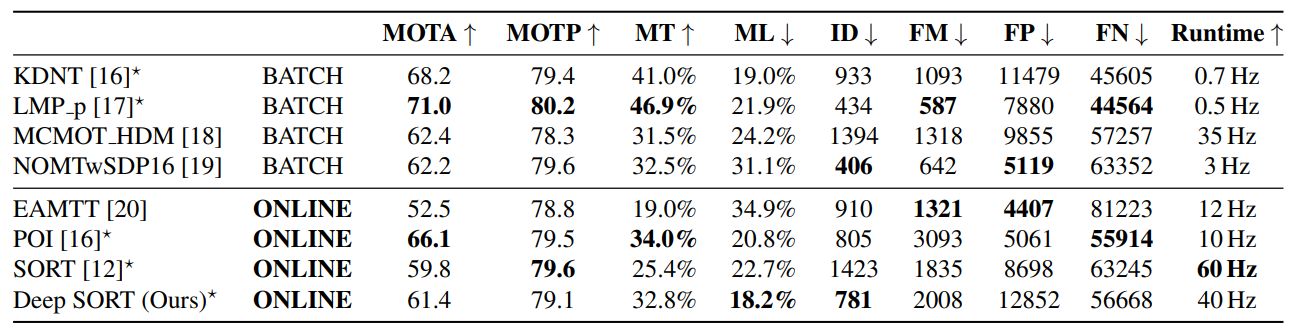## 4. 总结¶

Deep SORT可以看成三部分:

• 检测: 目标检测的效果对结果影响非常非常大, 并且Recall和Precision都应该很高才可以满足要求. 据笔者测试, 如果使用yolov3作为目标检测器, 目标跟踪过程中大概60%的时间都花费在yolov3上,并且场景中的目标越多,这部分耗时也越多(NMS花费的时间).
• 表观特征: 也就是reid模型,原论文中用的是wide residual network,含有的参数量比较大,可以考虑用新的、性能更好、参数量更低的ReID模型来完成这部分工作。笔者看到好多人推荐使用OSNet，但是实际使用的效果并不是特别好。
• 关联：包括卡尔曼滤波算法和匈牙利算法。

JDE=YOLOv3和reid融合

FairMOT=CenterNet和reid融合